Kids Encyclopedia Facts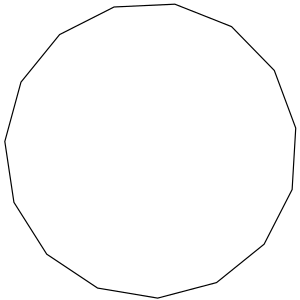Type Regular polygon
Edges and vertices 15
Schläfli symbol {15}
Coxeter diagram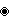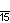Symmetry group Dihedral (D15), order 2×15
Internal angle (degrees) 156°
Dual polygon Self
Properties Convex, cyclic, equilateral, isogonal, isotoxal

A pentadecagon or quindecagon or 15-gon is a shape with 15 sides and 15 corners.

## Contents

All sides of a regular pentadecagon are the same length. Each corner is 147.27°. All corners added together equal 156°.

### Area

The amount of space a regular pentadecagon takes up is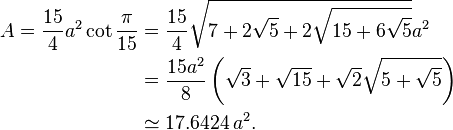\begin{align} A = \frac{15}{4}a^2 \cot \frac{\pi}{15} & = \frac{15}{4}\sqrt{7+2\sqrt{5}+2\sqrt{15+6\sqrt{5}}}a^2 \\ & = \frac{15a^2}{8} \left( \sqrt{3}+\sqrt{15}+ \sqrt{2}\sqrt{5+\sqrt{5}} \right) \\ & \simeq 17.6424\,a^2. \end{align}

a is the length of one of its sides.

## Uses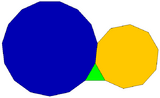A regular triangle, decagon, and pentadecagon can completely fill a plane vertex.

## Images for kidsPentadecagon Facts for Kids. Kiddle Encyclopedia.